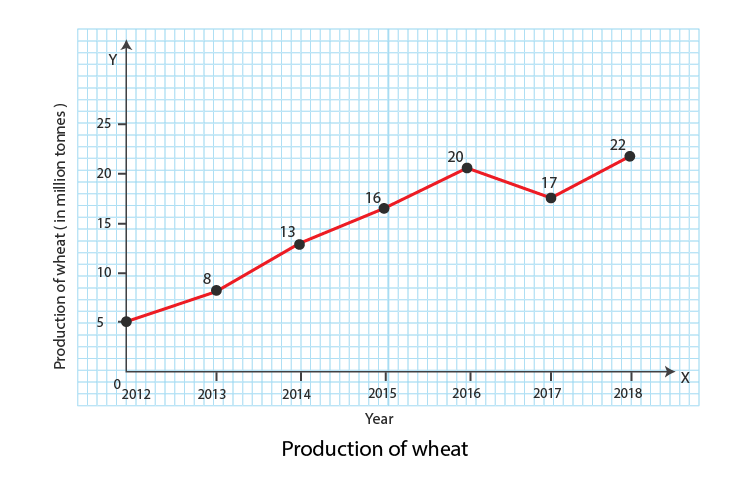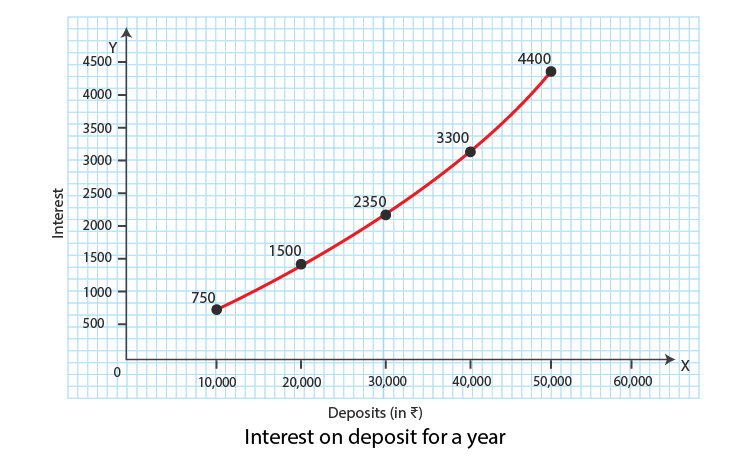# T.R. Jain and V.K. Ohri Solutions Class 11 Statistics Economics Chapter 8 - Arithmetic Line- Graphs or Time Series Graphics

T.R. Jain and V.K. Ohri Solutions for Class 11 Statistics for Economics Chapter 8 – Arithmetic Line- Graphs or Time Series Graphics is regarded as an important concept to be studied thoroughly by the students. Here, we have provided T.R. Jain and V.K. Ohri Solutions for Class 11.

 Board CBSE Class Class 11 Subject Statistics for Economics Chapter Chapter 8 Chapter Name Arithmetic Line- Graphs or Time Series Graphics Number of questions solved 02 Category T.R. Jain and V.K. Ohri

Chapter 8 – Arithmetic Line- Graphs or Time Series Graphics cover below-mentioned concepts

• Construction of graph
• The rule for constructing a graph

## T.R. Jain and V.K. Ohri Solutions for Class 11 Statistics for Economics Chapter 8 – Arithmetic Line- Graphs or Time Series Graphics

Question 1

Represent the following data (hypothetical data) graphically.

 Year 2010 2013 2014 2015 2016 2017 2018 Production of wheat (in million tonnes) 5 8 13 16 20 17 22

SolutionQuestion 2

Draw the graph of interest on deposit for a year.

 Deposits (in ₹) 10,000 20,000 30,000 40,000 50,000 Interest (in ₹) 750 1,500 2,350 3,300 4,400

SolutionQuestion 3

How many types of time series graph are there?

Solution:

There are two types of time series graph.

• One variable graph
• Two or more than two variable graphs

For more T.R. Jain and V.K. Ohri solutions, question papers, sample papers, syllabus, and commerce notifications, stay tuned to BYJU’S.

 Important Topics in Economics: